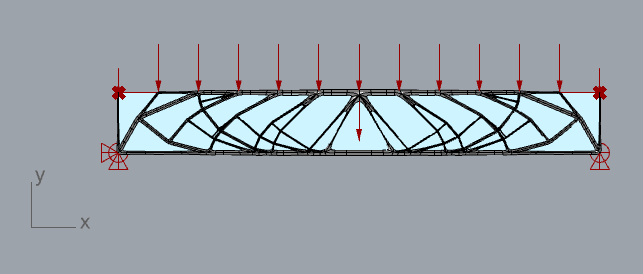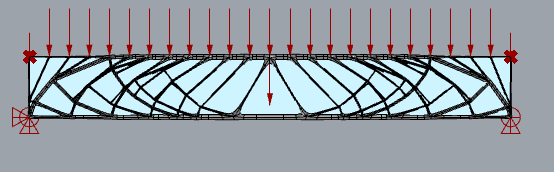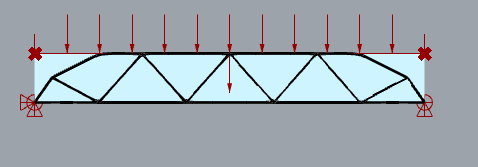# Input to force structure position in some locations?

Is there a way of inputting into Peregrine the positions where structure must exist?

E.g. I am using the Warren Truss example file as a starting point to look at optimising some arrangements where a truss has a continuous UDL applied to the top chord. How do I best tell Peregrine that I need the top chord to be continuous from end to end (i.e. in the optimisation, there must always be a member there as a minimum)?

I have tried inputting ‘UserNodes’ at the top left and top right domain bounds, which introduces a vertical element (see snip) but the top chord is not continuous across. This is understandable as the missing part of the top chord isn’t needed to resist the loads (since UDLs are converted to equivalent point loads rather than actually being UDLs).If I increase the divisions in my UDL load then the top chord extents become continuous in order for a member to be there wherever there is a converted point load (see snip). This achieves a continuous top chord but introduces a lot of additional members and therefore complexity due to the UDL division pointsIf I use the ‘Optimize Warren Truss’ geometry option then there are loads trying to apply to the top chord but there’s no member there. This code is before the ‘UserNodes’ input so doesn’t include the effect of the top left and top right point input.I would appreciate your thoughts, thanks!